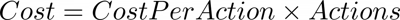Features

# Cost Per Action Calculator

Cost Per Action or CPA is a digital marketing measure of the cost of advertising taken for a user to make the desired action.

Cost per action (CPA) is a key success metric in performance marketing. It is a measure that shows the relative cost of a user taking the desired action such as filling out a lead form. Many digital marketing professionals have historically measured cost per action on a post click and a post impression or view-through basis. Using a post impression or view-through measurement can be used to make marketing metrics look better than they actually are when combined with retargeting.

## Cost Per Action Formula

### Calculate CPA

Cost per action or CPA is calculated by dividing the total cost by the number of actions.### Calculate Actions

To calculate the number of actions from the total cost and the cost per action, simply divide the total cost by the number of actions. Remember that if the CPA has been rounded in any way the number of actions may not be correct. It is not possible to have a fraction of an action.### Calculate Cost

To calculate the total cost from the number of actions and the cost per action, simply multiply the cost per action by the number of actions. Remember that this number may not be exactly correct if the cost per action has been rounded.